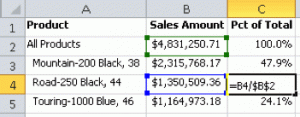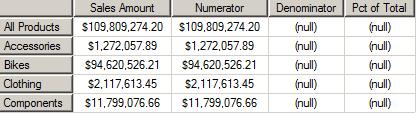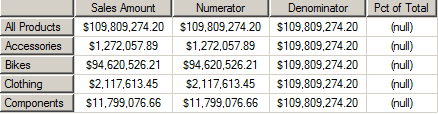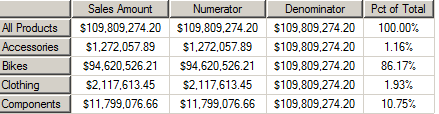After an intermission for the holidays, I’m resuming my series of posts that provide an introduction to MDX. This series began with Location, Location, Location, and continued through A Gentle Introduction to Sets. At this point, you should have a good idea of the basic structure of a query using objects in an Analysis Services cube. In this post, I’m going to explain how you can expand your options by adding objects at query time.

You might do this during cube development to test out calculation syntax before adding it to the cube, or you might do this only when you need to run a one-time query to answer a specific question or test the cube. You can even use this technique for Reporting Services reports or with  other tools. But I have to recommend that, wherever possible, you should put calculations inside a cube which would render moot what I’m about to show you.  Why? So you don’t have to reinvent the wheel each time you need a calculation. One of the reasons we build cubes is to centralize business logic, which includes calculations. That said, some calculations can’t go in the cube. Whatever your reason, there will likely come a time that you’ll need a calculation added to your query, so the point of this post is to prepare you for that time.

We’ll start with an analogy in Excel, using a percent of total calculation as shown below. The formula consists of a numerator to get the sales amount on the current row and a denominator that references the total sales amount in cell B2. We use the terms relative reference and absolute reference to describe the values in the numerator and the denominator, respectively. So for Road-250 Black, 44 on row 4, we have a formula =B4/\$B\$2, where B4 is a relative reference and \$B\$2 is the absolute reference. As we paste the formula into rows 2, 3, and 5, the formula changes to =B2/\$B\$2, =B3/\$B\$2, and B5/\$B\$2.We can get similar behavior in an MDX query by using tuples. I can create one tuple as an expression (the term we use in Analysis Services that corresponds to a formula in Excel) for the numerator, and a second tuple as an expression for the numerator. Let’s create a query that has a calculation for the numerator and denominator separately, and then a third calculation for the final calculation so that we can see the results independently.

First, I like to create the query with placeholders, just to get the query structure straight before I introduce anything else that might compromise the query as I add complexity. Not that this example is complex, but it’s a good habit to develop. Start simple, and build up from there. It’s too easy to mess yourself up with a stray comma or parenthesis. Note that we add in each expression in a WITH clause that precedes the SELECT statement, and that we add the expressions as measures. Then we can use them just like a measure that comes from the cube in the SELECT statement.

```WITH
MEMBER [Measures].[Numerator] AS NULL
MEMBER [Measures].[Denominator] AS NULL
MEMBER [Measures].[Pct of Total] AS NULL
SELECT
{[Measures].[Sales Amount],```
`[Measures].[Numerator],`
`[Measures].[Denominator],`
```[Measures].[Pct of Total]}
ON COLUMNS,
[Product].[Category].Members ON ROWS

```Now let’s update the numerator expression by replacing NULL with [Measures].[Sales Amount]. You can see below that the query result simply repeats the sales amount value from the first column in the second column. Let’s review what we know about tuples from Location, Location, Location. When the query resolves the tuple for the second column, the current measure – [Measures].[Numerator] – doesn’t really exist in the cube, but its expression redirects to [Measures].[Sales Amount] which then gets combined with the category member on each row to produce a tuple which in turn resolves as a value retrieved from the cube. Just like the tuples for each value in the first column. This tuple is like the relative reference we saw in the Excel example – Sales Amount varies according to the row.Next let’s update the denominator expression by replacing NULL with a tuple expression: ([Measures].[Sales Amount], [Product].[Category].[All Products]). This time the query results shown below repeat the tuple value that we see at the intersection of the first column and first row. When the query resolves the tuple for the third column, both the measure in the tuple and the category member come from the expression. Thus, we get a constant value for each row in the third column. This tuple is like the absolute value in the Excel example.So let’s ponder tuple behavior in query results again for a moment. Simply put, a tuple resolves as by combining the current column member, the current row member, and whatever members are in the WHERE clause (aka the slicer). If a member on rows, on columns, or in the WHERE clause is a calculated member, then whatever member the calculated member references in the expression will be overridden in the current row, column, or slicer member. If the expression is complex – that is, composed of operations on multiple tuples – then the contents of each tuple is evaluated independently. Thus, in the percent of total calculation, we have the numerator changing dynamically as row members change but the denominator remaining constant.

Putting the pieces together, we get the final query as shown below. Notice the FORMAT_STRING property added to the Pct of Total expression to get a percentage format. It’s not necessary for Numerator or Denominator because the use of Sales Amount directly (rather than as part of a computation) results in the use of the currency format string as defined for the measure in the cube.

```WITH
MEMBER [Measures].[Numerator] AS [Measures].[Sales Amount]
MEMBER [Measures].[Denominator] AS ([Measures].[Sales Amount],